```What products are involved in this article?
This article is based on the use of RCP (Rheonics Control Panel) connected to the SME (Smart Module Electronics) from SRV, SRD, DVP, and DVM. Hence it can be used for any of these Rheonics sensors```
```What is the purpose of this article?
Explain the process to get a temperature compensated viscosity with Arrhenius Model```

`1. Temperature-compensated Viscosity`

Viscosity, defined as the ratio of shear stress to shear rate, is an important fluid property that has an increasing presence in monitoring processes and control systems.
Viscosity is affected by temperature and pressure. For most liquids, the viscosity decreases with temperature, whereas it increases for gasses.
In most cases, we want to monitor the fluid viscosity at a constant reference temperature. If the process temperature isn’t constant, an additional source of error to the control system is added. To avoid the temperature dependence on the fluid’s viscosity and truly monitor its consistency, the temperature compensated viscosity is introduced.
To compensate for temperature effects, we need a mathematical model that can be based on
, Polynomial, or Arrhenius functions.

The Arrhenius model is used in nutrition, chemical, cosmetic and pharmaceutical industries, liquids viscosity parameters are essential for energy transference calculations and for hydraulic calculations of fluid transport. Also, is commonly used for pure liquids and solvents, binary Newtonian liquid mixtures.

`2. Math model`

The SRV and SME are together a powerful tool in the measurement of inline viscosity and temperature. With the use of mathematical models, temperature compensated measurements can be obtained.

Below is the mathematical formula for the viscosity Arrhenius model used in the RCP (Rheonics software).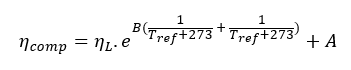Equation 1: Viscosity Arrhenius Model.

Where:

ηcomp - Compensated viscosity, calculated by the SME.

ηL - Live viscosity, read by the SME.

TL - Temperature, read by the SME.

Tref - Reference temperature, is the temperature around which the SME will compensate for the readings. This is defined and should be added by the user.

A - Compensation factor.

B - Compensation factor.

Along with the reference temperature, the reference viscosity (viscosity at Tref) is also of relevance for the calculations.

`3. How does this mathematical model work?`

The user needs to take viscosity readings at the normal operation temperature range, and store viscosity data also at higher and lower temperatures. Once enough data is obtained next steps can be followed to obtain the A and B parameters to input in Equation 1.

For this example, 30°C is used as the typical set temperature of this process line. The additional measurements extend a bit beyond the expected temperature range.

3.1. Table 1 shows the data points for this example, it includes viscosity and temperature measurements from the sensor. As mentioned before, we want to create a compensation at 30°C which is the temperature set in this process, at this temperature, the expected viscosity is 33.9 cP.

 Temperature [°C] Live Viscosity [cP] 60 9.42 50 13.6 40 20.3 30 33.9 20 60.1 10 116 0 255

Table 1. Data points from inline measurements.

3.2. Plot data points of Viscosity vs Temperature to study the behavior of the data. In this scenario, the data behavior can be represented by an exponential curve.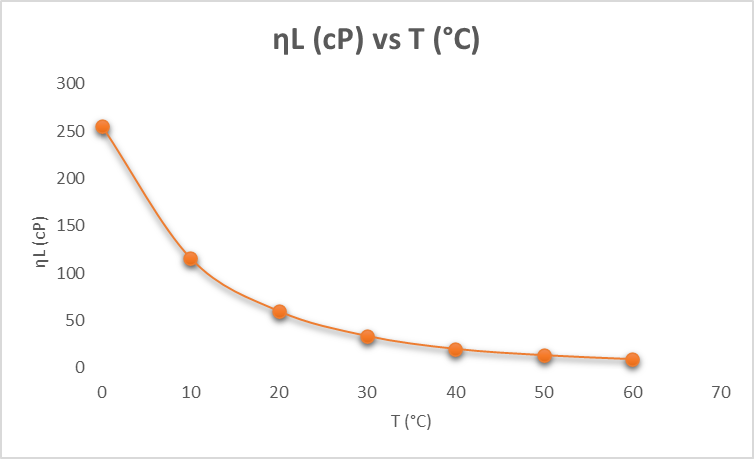Figure 1: Plot chart Live Viscosity vs Temperature.

3.3. To obtain coefficients A and B from equation 1, we will simplify the expression by using variables T' and Y', so we get the linear equation 2.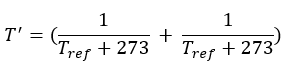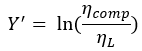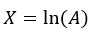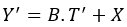Equation 2. Linearized Arrhenius model

3.4. Calculate Y' which is the natural logarithm of the compensated viscosity divided by the real-time viscosity measured by the sensor from our data points.

 Y'= Ln(Comp. Visco/Live Visco) @30 °C Results Y'= Ln(33.9/255) -2.0178 Y'= Ln(33.9/116) -1.2302 Y'= Ln(33.9/60.1) -0.5726 Y'= Ln(33.9/33.9) 0.0000 Y'= Ln(33.9/20.3) 0.0000 Y'= Ln(33.9/13.6) 0.9133 Y'= Ln(33.9/9.42) 1.2806

Table 2. Natural logarithm on the viscosity relation table.

3.5. Calculate T' that relates the ref temperature and measured temperature from our data points.

 T' = [(1/(Tref+273))-(1/(TL+273)) @30 °C Results T' = [(1/(30+273))-(1/(0+273)) -0.00023 T' = [(1/(30+273))-(1/(10+273)) -0.00011 T' = [(1/(30+273))-(1/(20+273)) 0.0000 T' = [(1/(30+273))-(1/(30+273)) 0.000105 T' = [(1/(30+273))-(1/(40+273)) 0.000204 T' = [(1/(30+273))-(1/(50+273)) 0.00123 T' = [(1/(30+273))-(1/(60+273)) -0.00036

Table 3. Variation of the reference temperature and measured temperature.

3.6. Plot the T' vs Y' and use an exponential trendline to find A and B values or make a linear regression analysis to find the coefficients.

 T' = [(1/(Tref+273))-(1/(TL+273)) @30 °C Y'= ln(V') -0.00023 -2.0178 -0.00011 -1.2302 0.0000 -0.5726 0.000105 0.0000 0.000204 0.5128 0.00123 0.9133 -0.00036 0.9133

Table 4. Data points for viscosity and temperature referenced to known values

A graphing tool is used to create a trendline with the points obtained, it can also show the trendline equation.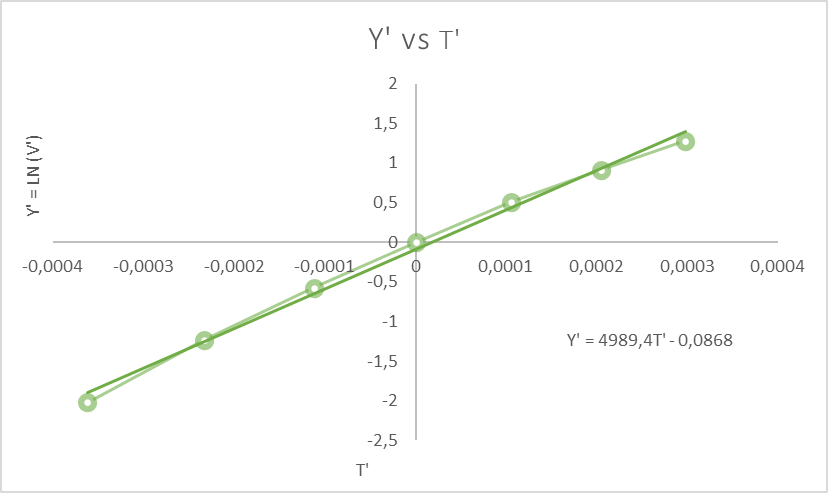Figure 2: Plot chart Y' vs T'.

As you can see we applied a linear trendline and the equation obtained is: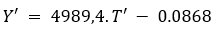Equation 3. Linear trendline

And this is compared with Equation 2 which is above in this document. As a result of this comparison, we get the following: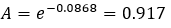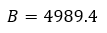`4. What do we expect to obtain with this model?`

We are looking for a constant measurement in the range of 33.9 cP which is the set parameter of viscosity. In this example, A is 0.917, and B is 4989.4, with all the input parameters set on the SME through the RCP software, the temperaturecompensated viscosity will look like the next graph in Figure 3.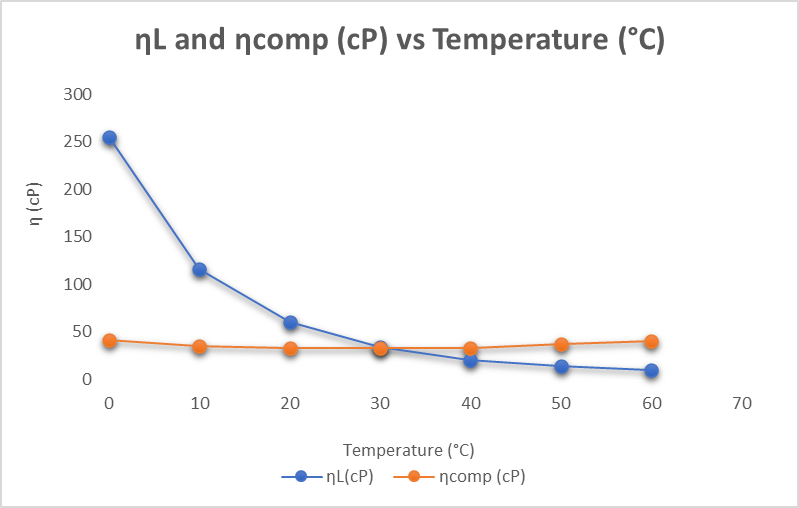Figure 3. Expected results when applying the mathematical model.

As we can see we obtain a linear behavior (Orange line) that represents the viscosity compensated to 30 °C with the Arrhenius model.

` 5. How to load models into the SME?`

Video 1. Configuration Arrhenius model in RPC Software.

`Resources`

It is also possible to see and use the calculated data through 4-20mA channels, Profinet, Modbus, and others. Follow this article on that: Using calculated data from SME